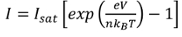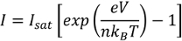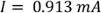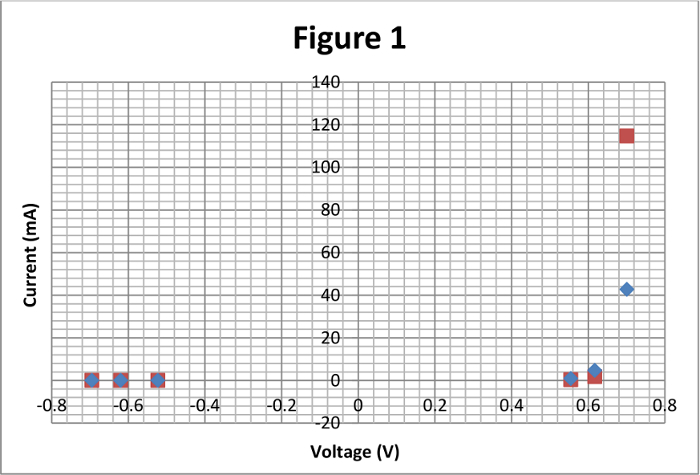Trial ends in

This content is Free Access.

Subtitles
Semiconductors

# Semiconductors

Article

### Overview

Source: Derek Wilson, Asantha Cooray, PhD, Department of Physics & Astronomy, School of Physical Sciences, University of California, Irvine, CA

Semiconductors are materials whose ability to conduct an electrical current depends strongly on their temperature and level of impurity. The most common type of semiconductor material is crystalline silicon. Most pure semiconductors are not outstanding conductors; to improve conductivity, a pure semiconductor is often combined or "doped" with an impurity. These impurities are either donors, like phosphorus and arsenic, that donate electrons to the silicon, or acceptors, like boron and aluminum, that steal electrons from the silicon. When acceptors take electrons from the silicon, they leave regions of positive charge called "holes" that effectively behave as positively charged electrons.

A p-type semiconductor is formed when doping makes holes that are the dominant charge carrier in the material. An n-type semiconductor is formed when a semiconductor is doped such that the dominant charge carrier is the electron. As one might expect, a p-n junction is formed at the boundary between the p-type semiconductor and n-type semiconductor. The interaction of electrons and holes at the junction gives rise to the remarkable behavior seen in circuit components such as diodes and transistors. This lab will explore the properties of a single p-n junction in the form of a semiconductor diode.

### Principles

At the junction between the p- and n-type materials, the electrons from the donor impurities in the n-type semiconductor combine with the holes from the p-type semiconductor. The donor impurity in the n-type semiconductor loses an electron and becomes a positive ion. The acceptor impurity in the p-type accepts this electron, forming a negative ion. The "depletion region" immediately surrounding the junction thus becomes deficient in either electrons and holes. In the depletion region, the n-type material region is now filled with positive ions, and the p-type material is dominated by negative ions. The positive ions repel electrons away from the n-type side of the junction, while the negative ions repel holes from the p-type side of the junction. The electric field from the build-up of ions at the p-n junction effectively prevents electrons or holes from flowing across the junction.

However, if a strong enough voltage is applied across the p-n junction, current can be made to flow again. If a positive voltage drop is placed across the junction (that is, a decrease in voltage from the p-type material to the n-type material), then the applied electric field may be able to overcome the force from the ions and can push electrons across the junction. The junction is said to be "forward-biased" in this case. Conversely, if a negative voltage drop is applied across the junction (that is, a decrease in voltage from the n-type material to the p-type material), then the applied voltage adds extra repulsion to the existing repulsion from the ions, and current cannot flow. In this configuration, the junction is "reverse-biased." Current can thus flow only in one direction through a p-n junction.

The Shockley diode equation describes the current,, flowing through a p-n junction as a function of its temperature and the voltage drop across it:(Equation 1)

where Isat is the saturation current typically in Amperes (A), e is the electron charge equal to 1.602 10-19 Coulombs (C), V is the voltage drop across the diode in Volts (V), n is a dimensionless parameter that varies from 1 to 2 and accounts for imperfections in the diode (n = 1 for an ideal diode), is Boltzmann's constant 1.38 10-23 m2 kg s-2 K-1, and T is the diode temperature in Kelvins (K). The saturation current is the tiny current that still manages to flow even when the diode is reverse-biased. One can see that the current grows exponentially for positive voltages and is exponentially dampened by negative voltages. There is also a strong temperature dependence. High temperatures decrease the current flow, and low temperatures cause the current to increase.

### Procedure

1. Observe the behavior of a p-n junction in the form of a semiconductor diode and measure its current-voltage characteristic curve.

1. Obtain a semiconductor diode, an LED (light-emitting diode), a power source, two digital multimeters, a 1 kΩ resistor, some banana cables and connectors, and a thermometer.
2. Look at the semiconductor diode. There should be a band on one of its ends. The side with the band is the "cathode". The side without the band is the "anode".
3. Make sure that the power supply is off before connecting any circuit components. Using the banana cables, connect the positive terminal of the power source to one side of the resistor, and the other side of the resistor to the anode of the diode. Then, connect a multimeter in ammeter mode to the cathode of the diode, and connect the other terminal of the ammeter to the negative terminal of the power source to complete the circuit.
4. Record the temperature in the room.
5. Set the power supply to generate a 5 V direct current and then turn it on.
6. Place the positive lead of the multimeter on the anode of the diode and the negative lead on the cathode.
7. In this configuration, the diode is considered forward-biased, so there should be a current flowing through the circuit, and the multimeter should display a voltage. Record the voltage and the current that the multimeters display.
8. Adjust the power supply to generate a different voltage. Record the voltage and current readings from the two multimeters, which should still be connected across and in series with the diode.
9. Repeat the previous step multiple times for a range of voltages. Record the ambient temperature during each repetition as well.
10. Remove the multimeter and turn the power supply off. While these voltages are not at a dangerous level, it is always safest to turn the power supply off when handling circuit components.
11. Keep all connections and setup the same, except flip the diode. The cathode is now connected to where the anode was previously connected, and vice versa for the anode.
12. Turn the power supply back on and reconnect the multimeter across the diode, with the positive lead of the multimeter on the anode of the diode and the negative lead on the cathode.
13. Record the new voltage and current that are displayed. It may be necessary to adjust the sensitivity of the multimeter. The diode is now reverse-biased, so only a miniscule current will be allowed to flow through the circuit.
14. Use the Shockley diode equation to calculate the current passing through the diode as a function of the voltage across the diode and the diode temperature. Assume that isat = 4 10-10 A.
15. Turn off the power supply and swap the diode for an LED.
16. The LED will have two pins. The longer pin is the anode, and the shorter pin is the cathode. Observe the LED in forward-biased and reverse-biased configurations.
17. Note that, since current only flows when the LED is forward-biased, the LED will only light up when in the forward-biased configuration and will be dark in the reverse-biased configuration.

Semiconductors are extensively used to build electronics and are the foundation of the global semiconductor industry.

A semiconductor is a solid substance that typically has conductivity between that of an insulator and that of most metals like copper or gold. The most common type of semiconductor material is crystalline silicon, which is made available in the form of thin, polished wafers.

There are two main types of semiconductors, p-type and n-type. These are fabricated next to each other and in different configurations to build semiconductor devices like p-n junctions or p-n-p transistors. Each configuration possesses unique electrical properties useful in different electronic devices.

This video will present the basic principles of semiconductor materials and properties of p-n junctions in the form of a diode. Next, it will illustrate a step-by-step protocol to characterize a diode, followed by some real-world applications of semiconductors.

Most pure or intrinsic semiconductors, like silicon, are not outstanding electrical conductors. This is because each silicon atom has four electrons in its valence or outermost shell. It shares these electrons with neighboring silicon atoms to form covalent bonds, creating a lattice structure devoid of free electrons. Thus a semiconductor is made more conductive by addition of impurities, a process also known as doping, to form doped or extrinsic semiconductors.

These impurities are of two types: donors and acceptors. "Donors", like phosphorus and arsenic, have five electrons in their valence shell. Four of these are used to form covalent bonds with adjacent silicon atoms. The remaining one electron is then free to move through the lattice. This type of doped semiconductor, in which electrons are the dominant charge carriers, is called an n-type semiconductor.

Now if the impurity is an acceptor molecule, like boron or aluminum, the result is different. These acceptors have only three electrons in their valence shell. Therefore, when an acceptor atom forms bonds with the surrounding silicon atoms, it leaves behind a region of positive charge called a "hole" that effectively behaves as a positively charged electron. The hole is now free to move through the lattice. This type of doped semiconductor, in which holes are the majority charge carriers, is called a p-type semiconductor.

Now, when a region on a single semiconductor crystal or a wafer is doped with donor atoms, and an adjacent region is doped with acceptor atoms, a p-n junction is formed. The interface between the p- and the n-regions is called the junction boundary.

At the junction boundary, the excess electrons in the n-region diffuse towards the p-region, and simultaneously the excess holes in the p-region diffuse towards the n-region.

As a result of this diffusion, the donor atoms in the n-region become immobile positive ions, while the acceptor atoms in the p-region become immobile negatively charged ions. Thus, at the boundary between the p and n-regions, a "depletion region" that is deficient in mobile electrons and holes is formed.

The negative ions in the p-type depletion region repel the electrons that diffuse from the n-region to the p-region, while the positive ions in the n-type depletion region repel the holes that diffuse from the p-region to the n-region.

In other words, the electric field from the build-up of ions in the depletion region effectively blocks the current from flowing across the junction. However, current can be made to flow again by applying a voltage across the intersection.

If a positive voltage drop is applied, also known as "forward-bias", the width of the depletion region reduces, decreasing the region's electric field, due to which electrons and holes jump across the junction, and thus current flows through the configuration.

Conversely, if a negative voltage drop is applied across the junction, known as "reverse-bias", then the depletion region width increases. This in turn increases the region's electric field strength and resistance to flow of electrons and holes across the junction.

Current thus flows only in one direction through a p-n junction. The Shockley diode equation can be used to calculate this current as a function of the diode's voltage drop and temperature. Here, 'e' is the electronic charge, 'n' is an ideality factor that characterizes how a real diode performs relative to an ideal diode, 'Kb' is the Boltzmann's constant, and 'Isat' is the small leakage current that flows through the device even when it is reverse biased.

Having completed the basics, let us now review a step-by-step protocol to characterize a p-n junction. First obtain the necessary materials and instruments, namely a semiconductor diode, a light-emitting diode or LED, a power source, two digital multimeters, a 1 kilo-ohm resistor, some banana cables and connectors, and a thermometer.

Look at the semiconductor diode and verify that there is a red terminal and a black terminal. The black terminal is called the cathode and the red terminal is the anode.

Next, connect the resistor in series with the anode of the diode. Then, using the banana cables, connect the positive terminal of the power source to the unconnected end of the resistor. Next connect the cathode of the diode to the positive terminal of an ammeter and the ammeter's negative terminal to the negative terminal of the power source to complete the circuit to complete the circuit.

The diode is now forward-biased. Record the temperature of the room. Next, set the power supply to feed +5 volts direct current through the circuit.

As the diode is forward-biased, there should be a current flowing through the circuit, and a voltage drop across the diode.

Next, connect the positive lead of a second multimeter on the anode of the diode, and the negative lead on the cathode. Ensure the multimeter is in voltmeter mode and measure the voltage drop. Also, note the current as recorded by the ammeter.

Now, adjust the power supply to generate a different voltage and record the corresponding drop across the diode using the voltmeter, and the current through it using the ammeter.

Also, note the ambient temperature for each reading, and repeat the protocol for a range of diode voltages.

Once all the measurements are recorded, disconnect the voltmeter and turn OFF the power supply. Keeping everything else the same, flip the diode so that the anode and cathode connections are now reversed and the diode is connected in the reverse bias mode.

Turn ON the power supply, and reconnect the voltmeter across the diode, with the positive lead of the multimeter connected to the anode of the diode and the negative lead to the cathode.

Record the voltage drop across the diode, the temperature and the current flowing through the diode for a range of diode voltages. Turn off the power supply and disconnect the diode.

Lastly, connect an LED in place of the diode, and observe the LED in both forward and reverse bias configurations for a range of voltage drops.

With the protocol now complete, let's review the results of both the forward and reverse-bias experiment using both the diode and the LED. First, calculate the current passing through the diode for the various voltage drops using the Shockley diode equation and the manufacturer provided Isat value. For example, for a temperature of 293 kelvin and a measured diode voltage of 555 milli-volts, the current through the diode can be calculated to be 0.913 milli-amperes.

Typical results for the circuit measurements with the diode connected in forward and reverse bias are listed in the table. The calculated and measured current is plotted as a function of the measured diode voltage. This is called the "Characteristic curve" of the diode.

The plot shows the exponential dependence of both the measured and calculated currents on the diode voltage. Specifically, it is observed that when the diode is forward biased, it allows the current to flow through.

But when the diode is reverse biased, no current flows through it, effectively making it a valve that only permits current flow in one direction. The tiny current that still manages to flow, even when the diode is reverse-biased, is the saturation current.

Semiconductors form the basis of the entire electronics industry ranging from the simple LEDs used in our television displays to the complex super-computers used for scientific data handling purposes.

Semiconductors are used to not only build p-n junctions or diodes, but also transistors, which are n-p-n or p-n-p junctions. These transistors are the basis of all modern electronics, as they can be used to construct logic gates, which are circuits that can perform basic Boolean logical operations such as AND, OR, NOT, and NAND. These logical operations can be combined as needed to perform more complex operations such as digital addition and multiplication. It can even be used to build computer processors and memory.

Semiconductor materials can also be used to generate light for application in optical electronics. For example, a light emitting diode or LED is a p-n junction that emits light when activated. When a suitable voltage is applied to it, electrons recombine with holes within the device, releasing energy in the form of light.

LEDs made from semiconductors are more energy-efficient light sources than the traditional incandescent bulb. Therefore, LEDs have found applications in environmental and task lighting, electronic displays and advanced communications technology.

You've just watched JoVE's introduction to semiconductors. You should now understand the basics of semiconductors and the principles, workings and characteristics of the p-n junction. Thanks for watching!

### Results

Typical results for the circuit measurements are shown in Table 1. The Shockley diode equation describes the current through a diode as a function of the temperature of the diode and the voltage drop across it. For a temperature of 293.0 K, a voltage of 555 mV across the diode, and an arbitrary (but representative) ideality factor of n = 1.5,The current through the diode is calculated for all of the measured voltages. The characteristic curve of the diode (current as a function of voltage) is plotted in Figure 1. The exponential dependence of the current on voltage is clearly seen. When in forward-biased, the diode allows current to flow. When in reverse-biased, only the microscopic saturation current can flow, effectively making the diode a valve that only permits current flow in one direction.

Table 1: Results.

 Measured Voltage (V) Measured Temperature (K) Measured Current (mA) Calculated Current (mA) 0.555 293.0 0.372 0.913 0.617 293.1 1.813 4.66 0.701 293.1 114.67 42.7 -0.523 293.2 0.0014 -4 * 10-7 -0.620 293.0 0.0011 -4 * 10-7 -0.695 292.9 0.0008 -4 * 10-7Figure 1: Theoretical points from the Shockley diode equation are in blue. Measured data points are in red. An arbitrary ideality factor of n = 1.5 was used in the Shockley diode equation. The discrepancy between measured and theoretical values might disappear if the true ideality factor of the diode was known.

### Applications and Summary

This lab explored the properties of semiconductors and a p-n junction in the form of a semiconductor diode. A diode is a circuit component composed of one p-n junction. The characteristic curve of the diode was measured, and the diode was observed to conduct an electrical current in only one direction. An LED contains a special type of p-n junction that emits light in addition to conducting unidirectionally.

Semiconductors are used extensively in the electronics industry. Semiconductor diodes contain only a single p-n junction, while transistors are made from n-p-n and p-n-p junctions; that is, two p-n junctions directly next to each other. Semiconductor transistors are the basis of nearly all modern electronics. They can be used to construct logic gates, which are circuits that can perform basic Boolean logical operations such as AND, OR, NOT, and NAND. These logical operations can be combined to perform more complex operations such as addition and multiplication, and can even be used to build computer processors and memory. LEDs made from semiconductors are more energy-efficient light sources than traditional incandescent bulbs.

### Get cutting-edge science videos from JoVE sent straight to your inbox every month.X